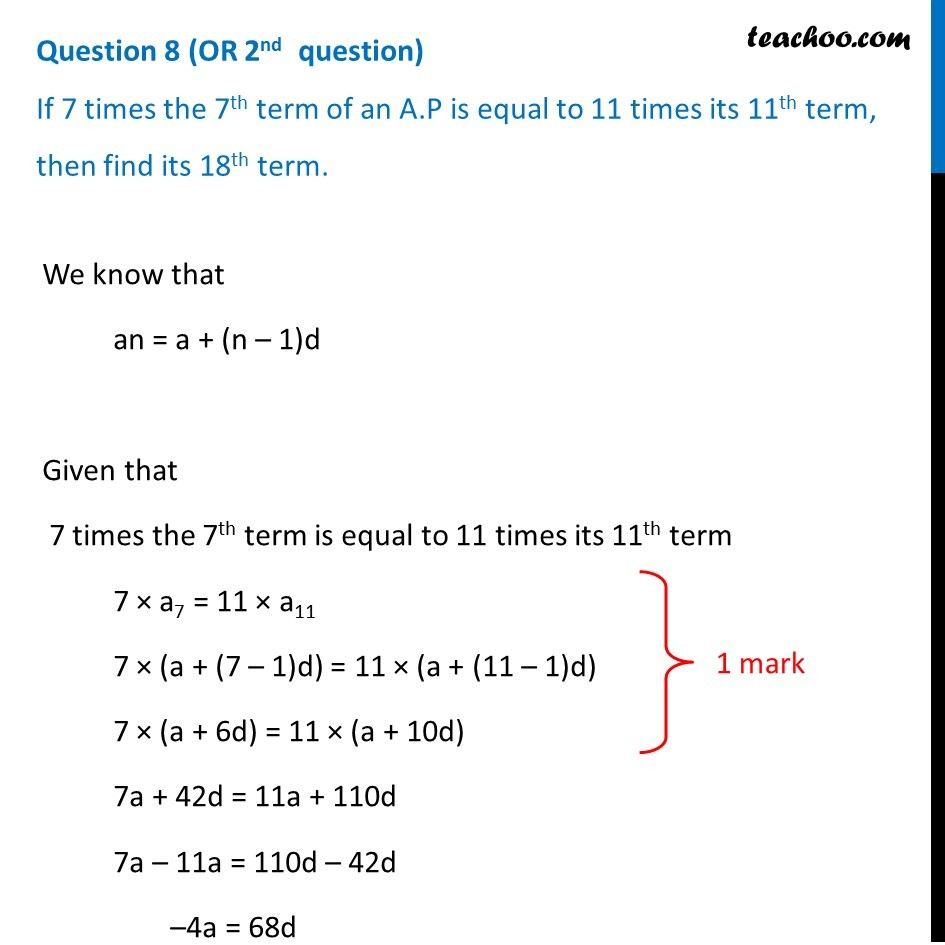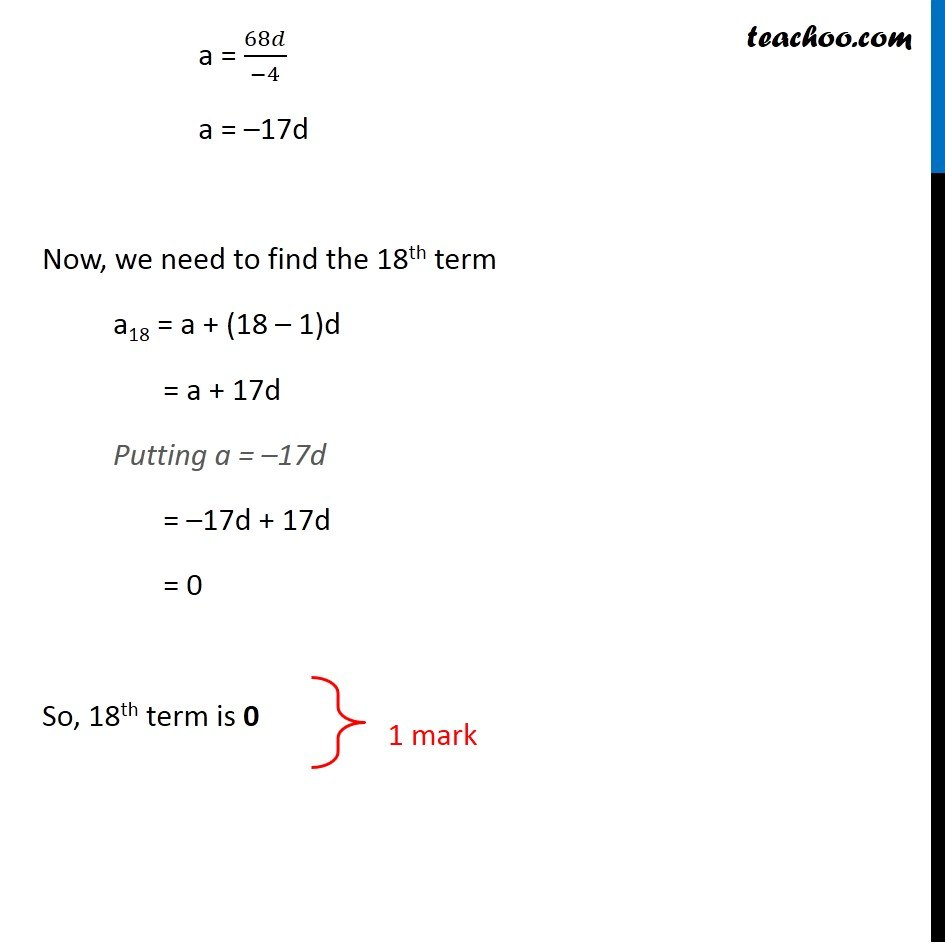CBSE Class 10 Sample Paper for 2019 Boards

Class 10
Solutions of Sample Papers for Class 10 Boards

Question 8 (OR 2 nd   question)

If 7 times the 7 th term of an A.P is equal to 11 times its 11 th term, then find its 18th term.Learn in your speed, with individual attention - Teachoo Maths 1-on-1 Class

### Transcript

Question 8 (OR 2nd question) If 7 times the 7th term of an A.P is equal to 11 times its 11th term, then find its 18th term. We know that an = a + (n – 1)d Given that 7 times the 7th term is equal to 11 times its 11th term 7 × a7 = 11 × a11 7 × (a + (7 – 1)d) = 11 × (a + (11 – 1)d) 7 × (a + 6d) = 11 × (a + 10d) 7a + 42d = 11a + 110d 7a – 11a = 110d – 42d –4a = 68d a = 68𝑑/(−4) a = –17d Now, we need to find the 18th term a18 = a + (18 – 1)d = a + 17d Putting a = –17d = –17d + 17d = 0 So, 18th term is 0